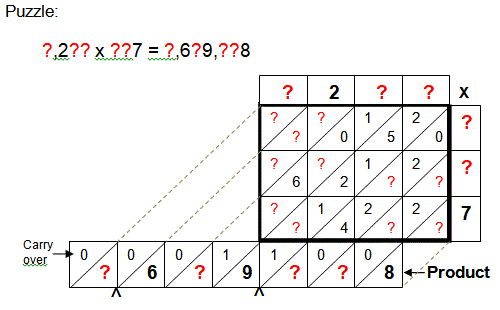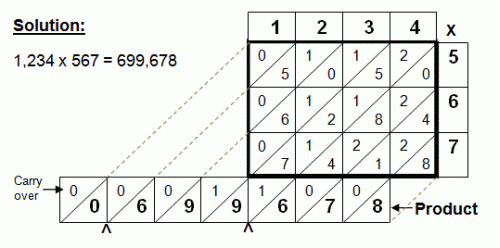Select PagePrintable version

Also known as a lattice method, the Chinese method provides an easy way to multiply any two numbers without the aid of a calculator.  In the following puzzles, some or part of the multiplied or added values are provided. The object of this puzzle is to find the missing values to determine the original numbers that when multiplied together gives you the correct product as shown on the bottom line.  See the method explanation before you start.

### Method explanation

The following example illustrates this process using a grid (sized to the numbers being multiplied) and slant lattice lines to form the product or answer.  As shown below, the four individual multiplication products (i.e., 7 x 5 = 35, etc.) are preformed and their two digits are divided by the lattice lines.  Adding the numbers along and within these diagonal lattice lines, the product in the example of 27 x 59 is determined to equal 1593, as shown. Using this simple illustrated method, the multiplication of any two numbers with any number of digits in each can be carried out. The number of grid boxes is determined by the number of digits in each number being multiplied (in example, matrix is 2 by 2).Find the missing values.### Solution## Feedback

There are more than one way of doing these puzzles and may well be more than one answer.  Please let me and others know what alternatives you find by commenting below.  We also welcome general comments on the subject and any feedback you’d like to give.   If you have a question that need a response from me or you would like to contact me privately, please use the contact form.

## Get more puzzles!

If you’ve enjoyed doing the puzzles, consider ordering the book; 150+ of the best puzzles in a handy pocket sized format. Click here for full details.